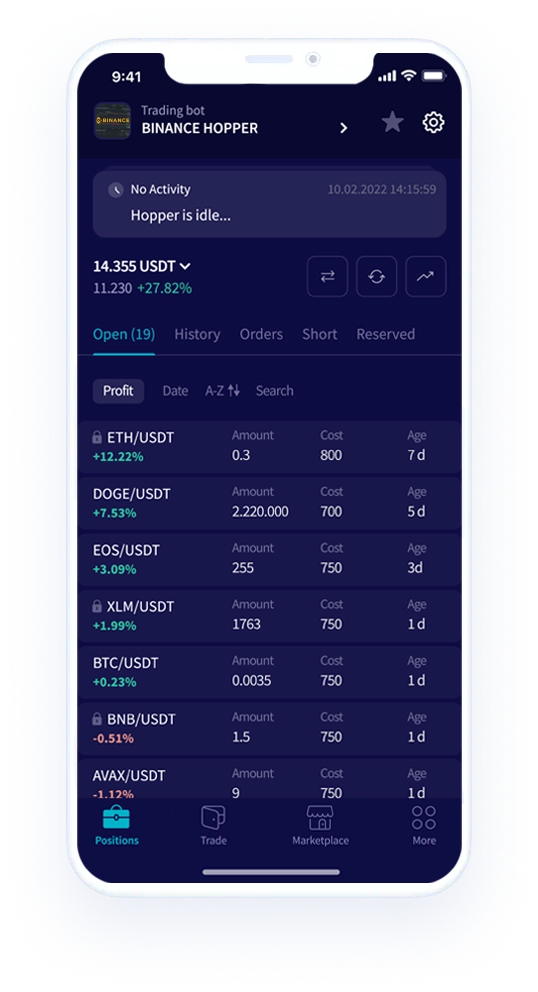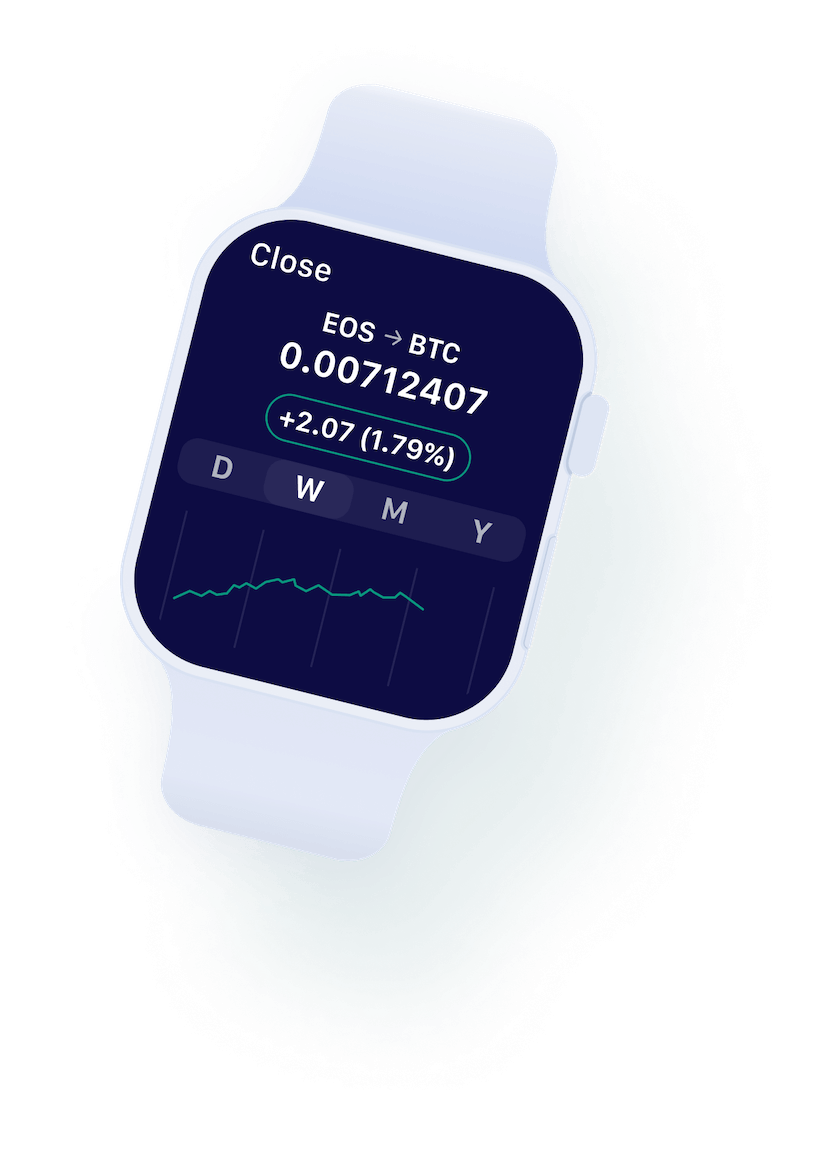0%# How To Calculate Cryptocurrency Profits

Best practices and helpful tools for calculating the profits from your cryptocurrency investments.

## How to Calculate Cryptocurrency Profits?

Profits in digital assets can accumulate in a variety of ways, from long-term investments to trading, scalping, and mining. Calculating crypto profits is simple math and relatively easy. However, there are certain factors that you need to consider before you calculate the gains and losses. This article discusses how to calculate cryptocurrency profits and helpful tools for tracking your investments.

## Simple Mathematics

Calculating cryptocurrency profits is not rocket science. It follows the basic concept of calculating gains and losses in mathematics. However, before calculating the final profit, you need to consider other factors, such as the current market price of the cryptocurrency, exchange fees, gas fees, investment charges, etc.

Now, let’s review how to calculate cryptocurrency profits. Generally, you use a fiat currency like the dollar or Euro to calculate the profits.

Say, for example, you bought 2 Bitcoins (BTC) for \$20,000 and sold them for \$40,000. The profits will be the difference between the selling price of Bitcoins and the cost price of Bitcoins.

Here, Selling price of 2 BTCs = \$40,000 Cost price of 2 BTCs = \$20,000 Profit = \$40,000 - \$20,000 = \$20,000

This is the simplest way to calculate cryptocurrency profits. But, please note that \$20,000 is the gross profit but not the net profit. To determine the net profit, you must deduct other associated costs incurred during the time of trading.

When you trade on a cryptocurrency exchange, you may incur trading fees. These fees are paid when you transfer cryptocurrencies to the customer or to the liquidity provider or conduct a transaction on the cryptocurrency exchange.

Therefore, while calculating net profit, you must deduct the exchange fees from the gross profit. For example, let’s say your transaction fee is 3%.

Total sales = \$40,000 Total cost = \$20,000 Gross profit = \$40,000 - \$20,000 = \$20,000 Transaction fees = 3% of total sales = 3% of \$40,000 = \$1200 Therefore, Net profit = Total profit – fees = \$20,000 - \$1200 = \$18,800

## Profit Calculators

Traders who invest in multiple cryptocurrencies often use crypto profit calculators to calculate gains and losses within minutes.

• Coinmash calculator – This straightforward calculator has an easy-to-use setup that can help you calculate your crypto profits within minutes. You have the amount you have invested and the currency you have used. Combine this with your bought token and the purchase date to finish the calculation. The results will show you the purchase price, current price, ROI, and present worth.

• Easy Bitcoin Calculator – Like the previous tool, Easy Bitcoin Calculator helps calculate profits and losses on Bitcoin investments. You have to enter the cost price, selling price, and the total number of Bitcoins, and unlike the Sabe calculator, you can also insert the fees incurred during the transaction. You can choose the long and short methods to calculate the profits.

## Calculating Crypto-to-Fiat Profits

While calculating the profits between crypto and fiat exchange, we must consider the exchange rate between the two currencies. Traders must decide how much to hold and when to sell the currencies to maximize profits. The transaction fees and network fees of the exchanges should also be considered before calculating the final profits. The exchange rates can be easily analyzed by using certain calculators:

• CryptoMarketCap Calculator – This tool allows you to calculate your cryptocurrency conversion into fiat currencies and vice versa. Enter the number of currencies, the fiat or cryptocurrency of your choice, select the currency you want to convert into, and the calculator will give you the price. You can convert BTC, BNB, XRP, ETH, DOGE, USDC, Polkadot, and many others into the fiat currency of your choice, including USD, AUD, ARS, ALL, BDT, BYN, and many more.

• Currexy – In this cryptocurrency converter, select a coin and convert it into USD, EUR, or other fiat currency. The tool allows you to view the currency charts and prices and compare them with other currencies. Enter the currency you want to exchange in the currency field, choose the conversion currency, and view the results immediately.

## Calculating Cryptocurrency Mining Profits

Bitcoin mining can be costly, especially due to the underlying costs associated with the mining process. To generate profits, the mining price must exceed the cost to mine Bitcoins or other cryptocurrencies.

To mine cryptocurrencies, miners incur operational costs such as electricity use and the costs to set up a computer system. The cost of electricity also depends on the geographic location of the miner. The total cost can also depend on hashing power, total consumption of watts, and pool fees for staking or mining cryptocurrencies. You can also use mining calculators to calculate the profits:

• BTC.com – Here, you can view the real-time mining pool hash rates. The mining pool calculator will give you the network hash rates, electricity costs, total cost, profit ratio, and estimated mining profits.

• CryptoCompare Calculator – CryptoCompare allows you to mine BTC, ETC, ETH, XMR, DASH, LTC, ZEC coins. You can calculate the profit ratio per day, mining cost, power consumption in watts, pool fees, and calculate the profit per day, week, month, or year.

## Calculating Profits on Crypto Taxes

Profits on cryptocurrencies, like any other income generated, are taxable. Though profits or incomes generated on cryptocurrencies are considered passive income, many government agencies, financial and tax collecting agencies consider them taxable income.

Your tax liability will depend on certain factors such as:

• The area where you reside. Taxes will be calculated based on the tax laws of the country.

• The amount of cryptocurrencies sold.

• The date of sale.

• The fiat value during the time of acquisition or sale.

Taxes will be levied on the profits earned on crypto assets. To calculate the profits, subtract the cost basis from the proceeds. For example, if your cost basis is \$5,000 and the proceeds are \$10,000, then, your profits are \$5,000 (proceeds minus cost basis). The tax will be calculated on the profits, i.e., \$5,000. Remember that only the net profits are taxable and not the entire proceeds or income.

The taxable amount also depends on how long a trader holds the crypto assets. The more you hold the cryptocurrencies, the less your taxes are. So, if you hold cryptocurrencies for longer periods, your taxes will be lower compared to short-term cryptocurrencies. So, it is always profitable to hold crypto assets for the long term. Note, taxes are only levied on profits, and traders do not have to pay any capital gains tax if they incur losses.

There are different types of crypto tax calculators available in the market, like the Crypto Trader Tax. These calculators pull information and transaction history from different mining pools and exchanges, calculate your overall capital gains or losses, and generate income and tax reports.

## Final Words

Cryptocurrency trading is immensely profitable but can be risky due to the unpredictable nature of the market. Hence, it is advisable that investors, especially new entrants, calculate the profits and losses diligently. Free online profit calculators can be handy and can help to calculate the profits within minutes. The results are accurate and are hassle-free.Get the weekly email with exclusive crypto analyses and news worth reading. Stay informed and entertained, for free.

World class automated crypto trading bot

Let's get startedRelated Articles### Bot Trading 101 | How To Apply a Scalping Strategy